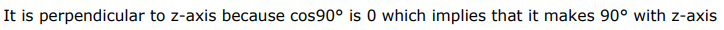# The straight lineQuestion:

The straight line $\frac{x-2}{3}=\frac{y-3}{1}=\frac{z+1}{0}$ is

A. parallel to the $x$-axis

B. parallel to the $y$-axis

C. parallel to the z-axis

D. perpendicular to the z-axis

Solution: